Scientific Calculator | Complex Number Calculator v1.3.2 (Paid) APK

Feature-Rich, Easy-to-Use Complex Number Calculator; A Scientific Calculator
Details
Title: Complex Number Calculator | Polar Complex Calc
Genre: Tools
Developer: A_M_I_R
Description

Use this complex number calculator as a scientific calculator. Any operation or function evaluation you can do with real numbers, you can also do the same with complex numbers by using this scientific calculator. Namely, calculate expressions containing real, imaginary and, in general, any complex number in any form including, but not limited to, the standard (rectangular) and polar forms.

You can enter complex numbers in the standard (rectangular) or in the polar form. The calculated values will be displayed in standard form; optionally, this scientific calculator displays the results in the polar form and other modular forms.

With a unique intuitive user interface this scientific calculator, being a comprehensive complex number calculator, computes arbitrary complicated complex number expressions with exceptional ease and converts complex numbers to polar form.

• Supports all standard mathematical functions with real, imaginary and complex numbers as their arguments including trigonometric, exponential, logarithmic, hyperbolic and inverse trig/hyperbolic functions plus the Gamma function, Γ, Psi function, Ψ and zeta function, ζ.

Swipe the number pad to the right to see more functions (trig, hyperbolic, inverses, etc.).

• Calculate expressions containing complex numbers in standard form a+bi and polar (phasor) form r∠(θ).

• Convert complex numbers from rectangular to polar and other modular forms and vice versa (long press i or ∠ to see all forms of the calculated result).

• RAD and DEG mode.
In addition to evaluating trig and related functions, this scientific complex number calculator allows the angle of polar form of complex numbers to be entered in radians or degrees.

• Fixed, scientific and engineering notations.

This scientific calculator also let you do statistics with one or two dimensional real data:
• x1, x2 ,x3, … .
• Press Σ to calculate sum, mean, max, min, variance, sample and standard deviation, median, upper and lower quartile, etc.

• x1, y1; x2, y2; x3, y3; … .
• Press Σ to calculate the equation of the linear regression line and graph it.

• Long press ! to calculate combinations C(n, r) and permutations P(n, r).

• Enter functions f(x) or parametric equations p(t) and generate table of values.

• Physical/Chemical/Atomic and other scientific constants.

• Easy to use unit converter (Time, Mass, Length, Velocity, and many more).

All of the above with more details are included in the built-in Instruction menu accompanying this scientific complex calculator.

Screenshots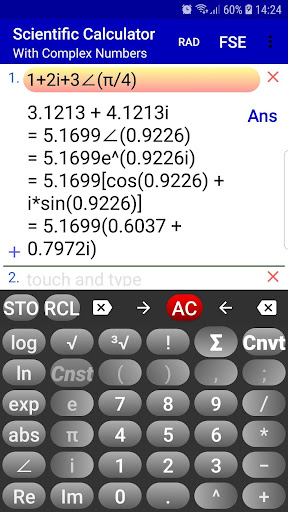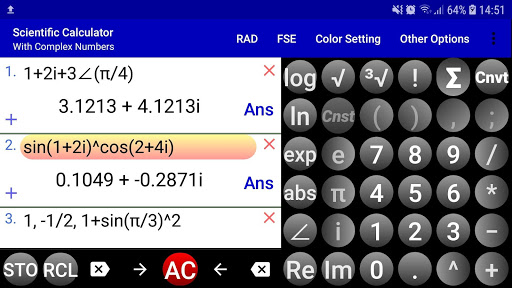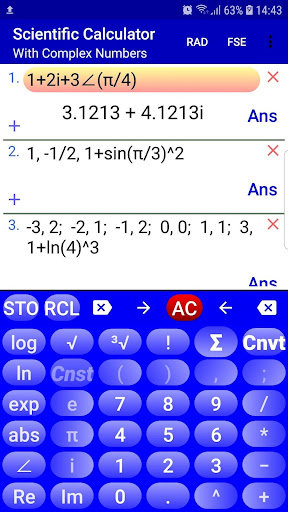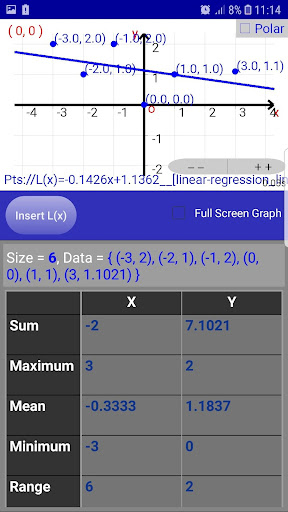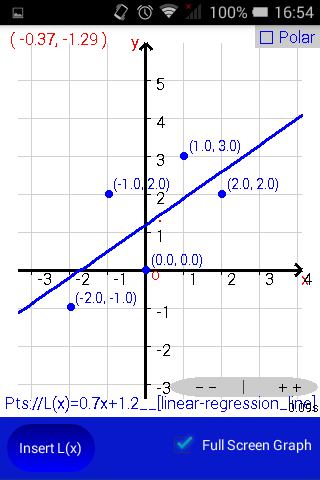Changelog

Crash fixes.
Performance improvement.

Rating and posting comments for this calculator on Google Play can help adding more features to this app.

User agreement: Please read the terms and conditions of using this app on https://sites.google.com/view/gcalcd/home/user-agreement

User guide is translated into more languages and, to keep the app size at the minimum, can be viewed on the developer web site.